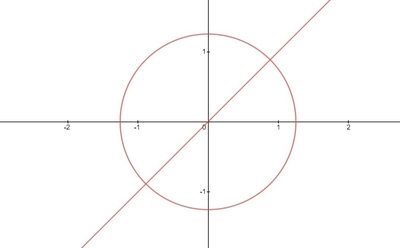# Let R be the region in the first quadrant inside the circle x^2 +y^2 = \frac{\pi}{2} and...

## Question:

Let {eq}R {/eq} be the region in the first quadrant inside the circle {eq}x^2 +y^2 = \frac{\pi}{2} {/eq} and below the line {eq}y = x {/eq}

a. Sketch the region

b. Use polar coordinates to evaluate the double integral {eq}\iint_R \cos(x^2 + y^2) \, dA {/eq}

## Application of Double Integrals:

Note that the double integrals formula for the area of the region is {eq}A=\int \int dydx {/eq} and to evaluate we need to find the limits based on the given bounding equations. Note also the conversion formulas from rectangular to polar and vice versa are the following {eq}r^{2}=x^{2}+y^{2},\:x=r\cos \theta ,\:y=r\sin \theta {/eq}.

## Answer and Explanation:

Below is the graph,First we need to find the limits, substituting the equation {eq}y=x {/eq} to the equation of the circel.

{eq}x^{2}+x^{2}=\frac{\pi }{2} {/eq}

{eq}2x^{2}=\frac{\pi }{2} {/eq}

Converting to polar coordinates using the following conversion formulas {eq}r^{2}=x^{2}+y^{2},\:x=r\cos \theta ,\:y=r\sin \theta {/eq}

Thus,

{eq}2r^{2}\cos ^{2}\theta =\frac{\pi }{2} {/eq}

Note that {eq}r=\sqrt{\frac{\pi }{2}} {/eq}

Then,

{eq}2\left ( \sqrt{\frac{\pi }{2}} \right )^{2}\cos ^{2}\theta =\frac{\pi }{2} {/eq}

{eq}\cos ^{2}\theta =\frac{1}{2} {/eq}

{eq}\cos \theta =\pm \frac{\sqrt{2}}{2} {/eq}

Since the region of concern in in the first quadrant thus we discard the negative.

{eq}\theta =\frac{\pi }{3} {/eq}

Thus, the limits are the following {eq}0\leq \theta \leq \frac{\pi }{3},\:0\leq r\leq \sqrt{\frac{\pi }{2}} {/eq}

Plugging-in the limits to the given integral expression,

{eq}\iint_R \cos(x^2 + y^2) \, dA=\int_{0}^{\frac{\pi }{3}}\int_{0}^{\sqrt{\frac{\pi }{2}}}\cos(r^2)rdrd\theta {/eq}

Integrate with respect to {eq}r {/eq}

{eq}\displaystyle =\int_{0}^{\frac{\pi }{3}}\left [ \frac{1}{2}\sin \left(r^2\right) \right ]^{\sqrt{\frac{\pi }{2}}}_{0}d\theta {/eq}

{eq}\displaystyle =\int_{0}^{\frac{\pi }{3}}\frac{1}{2}d\theta {/eq}

Integrate with respect to {eq}\theta {/eq}

{eq}\displaystyle =\frac{1}{2}\left [ \theta \right ]^{\frac{\pi }{3}}_{0} {/eq}

{eq}=\frac{\pi }{6} {/eq}

#### Learn more about this topic:Double Integrals: Applications & Examples

from AP Calculus AB & BC: Help and Review

Chapter 12 / Lesson 14
16K